### guitar hero 2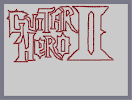Hover over the thumbnail for a full-size version.

Author hondaMXrider art author:hondamxrider game guitar hero n-art rated video 2007-09-15 4 by 5 people. \$guitar hero 2#hondaMXrider#none#00000000000000000000000000000000000000000000000000000000000000000000000000000000000000000000000000000000000000000000000000000000000000000000000000000000000000000000000000000000000000000000000000000000000000000000000000000000000000000000000000000000000000000000000000000000000000000000000000000000000000000000000000000000000000000000000000000000000000000000000000000000000000000000000000000000000000000000000000000000000000000000000000000000000000000000000000000000000000000000000000000000000000000000000000000000000000000000000000000000000000000000000000000000000000000000000000000000000000000000000000000000000000000000000000000000000000000000000000000000000000000000000000000000000000000000000000000000000000000|12^56,66!12^57,69!12^57,71!12^50,63!12^55,67!12^59,67!12^63,68!12^68,68!12^71,68!12^74,68!12^76,68!12^72,69!12^68,70!12^65,72!12^63,72!12^68,73!12^73,73!12^75,73!12^75,71!12^80,71!12^85,71!12^88,71!12^92,70!12^83,69!12^86,69!12^90,67!12^94,64!12^97,60!12^99,57!12^101,63!12^106,65!12^110,68!12^113,69!12^112,70!12^104,70!12^100,70!12^97,70!12^102,67!12^104,67!12^113,69!12^115,69!12^118,69!12^127,69!12^130,69!12^132,69!12^131,70!12^126,70!12^121,70!12^120,70!12^131,67!12^136,67!12^139,67!12^164,83!12^164,85!12^158,82!12^155,80!12^149,76!12^144,73!12^140,70!12^157,77!12^152,77!12^141,74!12^137,74!12^165,88!12^164,88!12^162,90!12^159,92!12^154,93!12^148,96!12^146,99!12^144,102!12^169,87!12^55,71!12^55,71!12^55,78!12^55,79!12^55,81!12^55,84!12^55,88!12^55,93!12^55,99!12^55,107!12^54,112!12^54,95!12^53,100!12^49,102!12^46,106!12^44,107!12^47,107!12^54,114!12^56,120!12^56,121!12^50,107!12^49,107!12^51,116!12^49,115!12^45,112!12^43,110!12^57,119!12^57,122!12^57,123!12^57,124!12^57,126!12^57,132!12^57,138!12^57,141!12^58,135!12^58,138!12^58,144!12^58,149!12^58,156!12^58,164!12^58,174!12^58,177!12^58,182!12^58,179!12^58,173!12^58,171!12^58,158!12^58,153!12^59,182!12^59,178!12^60,169!12^59,167!12^58,183!12^58,183!12^58,184!12^58,186!12^58,188!12^58,191!12^58,195!12^58,201!12^58,206!12^58,209!12^58,212!12^58,215!12^58,205!12^58,198!12^58,217!12^58,218!12^58,221!12^58,216!12^59,211!12^62,208!12^66,202!12^70,198!12^72,196!12^74,195!12^74,194!12^75,193!12^80,193!12^85,193!12^90,193!12^96,193!12^100,193!12^104,193!12^108,193!12^111,193!12^114,193!12^117,193!12^121,193!12^122,193!12^122,193!12^122,194!12^122,198!12^122,201!12^120,215!12^122,206!12^122,206!12^100,243!12^100,242!12^99,245!12^98,247!12^95,249!12^92,252!12^89,256!12^86,258!12^83,260!12^83,262!12^112,263!12^112,269!12^112,274!12^112,278!12^112,278!12^112,278!12^112,280!12^112,283!12^112,285!12^112,289!12^112,294!12^112,298!12^112,303!12^112,307!12^112,309!12^112,313!12^113,312!12^113,317!12^113,319!12^113,321!12^113,323!12^113,327!12^113,330!12^113,330!12^113,333!12^113,339!12^110,346!12^105,353!12^100,359!12^96,364!12^94,366!12^97,362!12^98,357!12^102,354!12^106,349!12^109,344!12^112,340!12^113,335!12^93,367!12^88,369!12^86,370!12^86,370!12^93,370!12^100,370!12^106,370!12^111,370!12^115,370!12^116,370!12^118,370!12^121,370!12^125,370!12^127,370!12^130,370!12^132,370!12^135,370!12^137,370!12^139,370!12^142,370!12^145,370!12^147,370!12^150,370!12^153,370!12^156,370!12^158,370!12^159,370!12^163,370!12^165,370!12^161,369!12^159,367!12^156,363!12^153,359!12^155,358!12^155,357!12^153,354!12^152,350!12^152,346!12^152,341!12^152,335!12^152,332!12^152,329!12^152,327!12^152,321!12^151,319!12^151,319!12^151,313!12^151,310!12^161,310!12^165,310!12^169,310!12^168,311!12^168,313!12^168,316!12^168,319!12^168,324!12^168,330!12^168,335!12^168,338!12^168,338!12^168,348!12^168,350!12^168,352!12^168,356!12^166,359!12^166,360!12^168,360!12^169,360!12^168,347!12^168,344!12^168,339!12^171,361!12^173,362!12^178,362!12^181,362!12^183,362!12^186,362!12^189,362!12^191,362!12^194,362!12^197,362!12^199,361!12^200,360!12^205,357!12^204,356!12^201,354!12^198,350!12^198,348!12^198,344!12^198,339!12^198,333!12^198,329!12^198,326!12^198,326!12^203,361!12^204,361!12^206,361!12^207,361!12^211,360!12^198,325!12^198,325!12^199,319!12^199,315!12^199,313!12^199,311!12^199,310!12^199,308!12^199,305!12^199,302!12^199,300!12^199,296!12^199,294!12^199,290!12^199,288!12^199,285!12^199,283!12^199,282!12^199,280!12^200,279!12^200,279!12^200,275!12^200,272!12^200,271!12^200,269!12^200,268!12^200,267!12^200,266!12^200,263!12^200,260!12^200,257!12^200,256!12^200,251!12^200,249!12^200,247!12^200,246!12^200,242!12^200,240!12^200,239!12^200,237!12^200,233!12^200,232!12^200,230!12^200,227!12^200,224!12^200,219!12^200,215!12^201,214!12^202,214!12^206,211!12^206,209!12^207,205!12^211,202!12^214,200!12^215,198!12^215,198!12^210,198!12^206,198!12^204,198!12^201,198!12^197,198!12^194,198!12^192,198!12^190,198!12^187,198!12^183,198!12^180,198!12^157,293!12^161,289!12^163,287!12^165,285!12^168,283!12^169,281!12^170,281!12^170,276!12^170,270!12^170,264!12^170,257!12^170,253!12^170,249!12^170,245!12^170,242!12^170,240!12^170,237!12^170,232!12^170,224!12^170,221!12^170,217!12^170,215!12^170,211!12^170,207!12^170,203!12^170,201!12^170,198!12^170,195!12^171,196!12^173,196!12^176,196!12^179,196!12^171,264!12^171,260!12^171,227!12^155,290!12^94,250!12^98,246!12^101,244!12^156,308!12^110,260!12^110,260!12^107,260!12^104,260!12^101,260!12^98,260!12^95,260!12^88,260!12^87,260!12^86,260!12^85,260!12^85,260!12^122,213!12^122,211!12^122,212!12^120,216!12^118,217!12^116,219!12^116,220!12^115,222!12^114,224!12^109,229!12^108,231!12^106,233!12^104,234!12^103,236!12^102,238!12^101,240!12^112,225!12^110,258!12^114,258!12^118,258!12^120,256!12^125,252!12^126,250!12^127,249!12^129,246!12^129,243!12^130,240!12^131,238!12^131,235!12^132,232!12^133,229!12^136,225!12^136,222!12^136,218!12^136,218!12^138,214!12^139,211!12^139,208!12^139,205!12^139,202!12^139,200!12^139,197!12^139,193!12^139,189!12^152,289!12^152,288!12^152,283!12^152,278!12^152,276!12^152,273!12^152,270!12^151,266!12^149,264!12^149,262!12^149,261!12^148,257!12^148,255!12^148,255!12^148,248!12^148,244!12^147,240!12^146,236!12^145,232!12^144,230!12^143,226!12^143,225!12^143,223!12^142,217!12^141,213!12^139,185!12^139,185!12^139,184!12^139,183!12^139,181!12^139,179!12^139,176!12^139,175!12^139,174!12^139,172!12^139,171!12^139,168!12^139,165!12^139,164!12^139,163!12^139,162!12^139,160!12^139,159!12^139,158!12^139,156!12^139,155!12^140,153!12^142,150!12^142,147!12^144,145!12^146,142!12^147,141!12^148,139!12^145,139!12^140,139!12^137,139!12^132,139!12^128,139!12^125,139!12^124,139!12^121,139!12^120,139!12^119,139!12^118,139!12^117,139!12^114,142!12^115,143!12^115,147!12^115,149!12^116,150!12^119,153!12^120,156!12^121,159!12^121,165!12^121,170!12^119,176!12^112,176!12^106,176!12^100,176!12^97,176!12^94,176!12^91,176!12^91,175!12^91,174!12^89,169!12^87,164!12^86,159!12^86,154!12^86,152!12^86,147!12^86,144!12^86,140!12^86,136!12^86,130!12^86,124!12^86,118!12^86,115!12^86,109!12^86,104!12^86,98!12^86,98!12^87,98!12^87,93!12^89,91!12^94,90!12^99,89!12^102,90!12^104,92!12^107,96!12^109,100!12^111,106!12^111,111!12^111,113!12^145,99!12^145,102!12^145,105!12^144,108!12^144,112!12^144,115!12^144,117!12^141,118!12^135,118!12^129,118!12^125,118!12^122,118!12^120,118!12^117,118!12^115,118!12^145,108!12^145,109!12^147,110!12^148,111!12^151,111!12^153,111!12^155,111!12^158,113!12^158,113!12^158,113!12^160,114!12^161,115!12^162,117!12^163,119!12^167,122!12^168,125!12^171,128!12^171,131!12^171,136!12^173,140!12^172,138!12^172,141!12^172,144!12^172,147!12^172,148!12^172,149!12^172,151!12^172,156!12^172,158!12^172,161!12^172,165!12^172,168!12^172,169!12^172,172!12^172,175!12^174,172!12^173,174!12^173,176!12^175,176!12^181,177!12^186,180!12^189,181!12^192,181!12^194,182!12^198,183!12^201,184!12^204,185!12^203,183!12^205,184!12^208,186!12^211,188!12^214,188!12^216,188!12^219,188!12^219,186!12^217,183!12^215,180!12^214,177!12^214,172!12^214,167!12^214,161!12^214,157!12^214,152!12^214,150!12^214,143!12^214,142!12^214,141!12^213,142!12^213,139!12^213,136!12^213,134!12^213,134!12^213,133!12^213,131!12^213,128!12^213,126!12^213,122!12^213,116!12^213,111!12^214,105!12^218,96!12^220,95!12^221,93!12^224,91!12^226,88!12^226,88!12^224,89!12^219,91!12^218,93!12^218,96!12^217,100!12^215,104!12^214,108!12^226,86!12^224,87!12^219,87!12^215,87!12^213,87!12^210,87!12^206,87!12^202,87!12^200,87!12^199,87!12^196,87!12^195,87!12^195,89!12^200,91!12^201,97!12^201,105!12^201,109!12^146,101!12^148,101!12^151,101!12^157,101!12^158,101!12^159,101!12^161,101!12^164,101!12^166,101!12^168,102!12^172,103!12^175,105!12^178,108!12^178,109!12^180,118!12^181,125!12^183,131!12^184,136!12^184,141!12^184,141!12^184,143!12^184,148!12^184,150!12^184,154!12^184,158!12^187,161!12^190,163!12^193,164!12^196,164!12^198,163!12^198,157!12^198,152!12^198,148!12^198,145!12^198,141!12^198,137!12^198,133!12^198,126!12^198,126!12^199,119!12^199,115!12^199,108!12^199,105!12^199,99!12^199,94!12^199,93!12^184,135!12^184,131!12^184,128!12^183,123!12^182,118!12^181,115!12^180,111!12^180,109!12^231,126!12^231,124!12^229,120!12^226,116!12^222,115!12^219,114!12^219,114!12^225,112!12^231,112!12^237,112!12^241,112!12^245,112!12^248,112!12^251,112!12^254,112!12^257,112!12^259,112!12^259,112!12^255,114!12^255,115!12^253,120!12^250,124!12^250,126!12^250,132!12^231,127!12^231,129!12^231,133!12^231,137!12^231,141!12^231,146!12^231,152!12^231,154!12^231,154!12^231,155!12^231,160!12^231,162!12^231,162!12^231,162!12^231,162!12^231,164!12^231,166!12^231,171!12^227,174!12^221,178!12^219,180!12^223,180!12^228,180!12^233,180!12^236,180!12^239,180!12^241,180!12^242,180!12^243,180!12^246,180!12^250,180!12^253,180!12^255,180!12^256,180!12^256,180!12^254,178!12^250,175!12^248,170!12^248,166!12^248,161!12^248,157!12^248,154!12^248,149!12^248,144!12^248,140!12^249,136!12^249,136!12^249,136!12^171,195!12^169,195!12^168,195!12^167,194!12^166,193!12^269,185!12^267,187!12^264,190!12^261,191!12^258,193!12^257,194!12^264,194!12^273,194!12^280,194!12^285,194!12^291,194!12^294,194!12^295,194!12^297,194!12^299,194!12^299,194!12^299,195!12^295,195!12^291,195!12^287,195!12^281,195!12^274,195!12^269,195!12^271,195!12^289,195!12^295,195!12^300,192!12^301,194!12^299,191!12^294,187!12^291,185!12^289,181!12^289,177!12^289,172!12^289,169!12^289,166!12^289,164!12^289,161!12^289,161!12^289,160!12^289,160!12^289,159!12^289,154!12^289,151!12^289,150!12^289,147!12^289,145!12^289,143!12^289,142!12^289,140!12^289,137!12^289,133!12^289,131!12^289,129!12^289,126!12^289,123!12^289,121!12^289,118!12^289,117!12^289,112!12^289,106!12^289,109!12^269,182!12^269,182!12^270,178!12^270,174!12^270,172!12^270,169!12^270,164!12^270,160!12^270,156!12^270,150!12^270,148!12^270,146!12^270,145!12^270,143!12^270,139!12^270,139!12^270,136!12^270,132!12^270,129!12^270,125!12^270,120!12^270,115!12^270,114!12^270,111!12^270,105!12^270,102!12^270,97!12^270,95!12^270,92!12^270,90!12^270,87!12^270,85!12^271,83!12^271,81!12^271,78!12^271,77!12^264,77!12^259,77!12^256,77!12^253,77!12^251,77!12^250,77!12^248,77!12^246,77!12^245,77!12^244,77!12^243,77!12^241,77!12^237,77!12^236,77!12^234,77!12^231,77!12^228,77!12^227,77!12^226,77!12^224,76!12^225,74!12^228,70!12^229,66!12^230,61!12^232,57!12^232,54!12^233,52!12^233,52!12^238,52!12^242,52!12^244,52!12^246,52!12^248,52!12^250,52!12^253,52!12^255,52!12^257,52!12^261,52!12^263,52!12^267,52!12^271,52!12^272,52!12^275,52!12^277,52!12^280,52!12^282,52!12^284,52!12^286,53!12^286,52!12^290,52!12^293,52!12^297,52!12^295,52!12^296,52!12^300,52!12^302,52!12^305,51!12^310,51!12^314,50!12^318,46!12^323,44!12^326,43!12^328,46!12^328,46!12^328,48!12^328,52!12^328,57!12^323,62!12^318,65!12^315,68!12^311,70!12^308,71!12^305,72!12^302,73!12^300,73!12^298,73!12^297,73!12^291,75!12^290,75!12^289,78!12^289,85!12^289,92!12^289,97!12^289,102!12^289,107!12^289,97!12^289,95!12^289,90!12^289,83!12^289,77!12^293,73!12^334,63!12^334,63!12^334,64!12^333,68!12^331,70!12^329,70!12^326,72!12^324,74!12^317,76!12^316,77!12^314,78!12^312,80!12^310,82!12^308,82!12^305,82!12^304,82!12^302,83!12^301,86!12^303,86!12^303,87!12^305,89!12^308,92!12^311,94!12^310,95!12^311,96!12^311,96!12^311,97!12^311,99!12^311,100!12^311,105!12^311,106!12^311,107!12^311,108!12^311,109!12^311,110!12^311,111!12^311,113!12^311,114!12^311,116!12^311,121!12^311,123!12^311,126!12^311,128!12^311,130!12^311,132!12^311,135!12^311,137!12^311,139!12^311,143!12^311,147!12^311,149!12^311,150!12^311,154!12^311,158!12^311,160!12^311,161!12^308,165!12^306,168!12^304,171!12^303,173!12^305,173!12^311,173!12^316,173!12^318,173!12^322,173!12^324,173!12^325,173!12^327,173!12^328,173!12^330,173!12^330,173!12^332,171!12^332,171!12^331,169!12^329,165!12^328,163!12^328,159!12^328,155!12^328,152!12^328,148!12^328,144!12^328,140!12^328,136!12^328,133!12^328,131!12^328,130!12^328,128!12^330,127!12^332,127!12^334,129!12^337,130!12^339,130!12^341,131!12^345,132!12^347,133!12^348,134!12^349,135!12^333,116!12^335,116!12^340,116!12^344,118!12^344,119!12^349,120!12^351,123!12^354,124!12^355,124!12^350,136!12^350,136!12^350,141!12^350,144!12^350,146!12^350,151!12^350,157!12^350,161!12^350,164!12^350,168!12^350,172!12^350,174!12^350,176!12^348,179!12^344,181!12^343,182!12^341,184!12^339,187!12^337,188!12^337,189!12^346,189!12^355,189!12^363,189!12^369,189!12^371,189!12^364,190!12^360,190!12^356,190!12^352,190!12^346,190!12^345,190!12^371,190!12^369,188!12^366,185!12^366,182!12^366,179!12^366,175!12^366,171!12^366,166!12^375,193!12^355,123!12^355,122!12^355,118!12^355,115!12^355,112!12^355,109!12^355,105!12^355,101!12^355,99!12^355,97!12^355,94!12^355,93!12^355,90!12^355,88!12^355,84!12^355,81!12^355,81!12^352,84!12^349,88!12^345,91!12^341,92!12^337,92!12^336,92!12^336,92!12^336,93!12^335,96!12^335,103!12^335,110!12^335,114!12^335,117!12^366,163!12^366,163!12^366,158!12^366,153!12^366,150!12^366,146!12^366,143!12^366,138!12^366,133!12^366,127!12^366,124!12^366,122!12^366,118!12^366,116!12^366,113!12^366,107!12^366,103!12^366,100!12^366,98!12^366,97!12^366,95!12^366,93!12^366,91!12^366,89!12^366,86!12^366,81!12^366,79!12^366,76!12^367,76!12^370,75!12^373,72!12^374,70!12^375,70!12^372,68!12^367,64!12^364,60!12^362,57!12^360,55!12^359,54!12^358,53!12^358,55!12^358,55!12^357,52!12^356,50!12^355,47!12^355,49!12^353,50!12^348,52!12^344,55!12^341,57!12^339,59!12^336,62!12^334,64!12^361,43!12^361,43!12^382,67!12^382,73!12^382,76!12^382,77!12^382,85!12^382,91!12^382,98!12^382,101!12^382,104!12^382,106!12^382,109!12^384,68!12^384,66!12^383,65!12^382,63!12^381,61!12^380,59!12^378,55!12^374,52!12^371,49!12^369,46!12^369,46!12^369,45!12^366,43!12^363,42!12^382,109!12^382,109!12^382,114!12^382,119!12^382,123!12^382,126!12^382,129!12^382,134!12^382,137!12^382,142!12^382,147!12^382,151!12^382,156!12^382,162!12^382,167!12^382,171!12^382,175!12^382,178!12^381,179!12^380,180!12^376,183!12^374,184!12^375,184!12^381,184!12^387,184!12^393,184!12^397,184!12^399,184!12^399,184!12^397,184!12^394,180!12^393,175!12^393,171!12^393,169!12^396,168!12^395,165!12^395,162!12^395,160!12^395,157!12^395,152!12^395,148!12^395,144!12^395,141!12^395,137!12^395,136!12^395,133!12^395,131!12^395,128!12^398,128!12^396,127!12^397,128!12^400,133!12^406,138!12^409,142!12^413,146!12^413,146!12^413,146!12^415,151!12^419,156!12^419,158!12^420,160!12^422,164!12^425,169!12^427,173!12^430,177!12^431,178!12^433,180!12^436,183!12^438,183!12^438,183!12^442,185!12^445,187!12^449,190!12^454,192!12^456,193!12^456,195!12^459,196!12^462,197!12^466,197!12^472,197!12^469,194!12^464,190!12^461,185!12^462,186!12^461,185!12^457,182!12^454,179!12^449,174!12^446,170!12^444,167!12^442,164!12^441,160!12^440,159!12^441,160!12^439,158!12^434,153!12^431,149!12^428,145!12^426,141!12^425,137!12^424,135!12^426,132!12^426,131!12^425,128!12^423,124!12^422,122!12^422,119!12^421,114!12^437,156!12^365,40!12^366,40!12^370,40!12^373,40!12^376,40!12^380,40!12^381,40!12^385,40!12^388,40!12^391,40!12^394,40!12^396,40!12^398,40!12^401,40!12^400,38!12^401,38!12^405,38!12^409,38!12^411,39!12^416,41!12^421,45!12^423,47!12^424,47!12^426,49!12^427,50!12^430,55!12^433,58!12^433,58!12^436,64!12^438,67!12^438,71!12^441,75!12^441,78!12^442,79!12^442,80!12^442,82!12^441,84!12^438,86!12^436,86!12^434,87!12^428,90!12^425,92!12^423,92!12^421,93!12^420,93!12^424,114!12^424,114!12^423,112!12^423,111!12^422,108!12^421,104!12^420,102!12^420,100!12^419,98!12^419,97!12^419,95!12^419,94!12^419,93!12^399,96!12^400,96!12^403,92!12^405,89!12^407,87!12^410,85!12^412,82!12^415,80!12^416,78!12^417,77!12^418,77!12^415,76!12^415,73!12^413,69!12^412,65!12^410,63!12^408,62!12^404,62!12^400,63!12^398,64!12^398,65!12^398,69!12^398,74!12^398,77!12^398,81!12^398,85!12^398,90!12^398,94!12^398,95!12^208,323!12^208,319!12^208,314!12^208,310!12^208,305!12^208,302!12^208,300!12^208,296!12^208,294!12^208,291!12^208,288!12^208,285!12^208,281!12^208,276!12^208,273!12^208,269!12^208,269!12^208,265!12^208,259!12^208,256!12^208,252!12^208,247!12^208,243!12^208,241!12^208,239!12^208,237!12^211,237!12^211,238!12^211,238!12^214,241!12^219,243!12^221,243!12^225,243!12^231,243!12^234,241!12^235,238!12^237,233!12^239,230!12^241,230!12^241,227!12^241,225!12^242,226!12^242,229!12^242,232!12^243,228!12^243,229!12^243,230!12^243,231!12^243,232!12^245,233!12^246,234!12^242,229!12^243,231!12^244,234!12^246,236!12^248,239!12^248,240!12^248,241!12^251,241!12^255,241!12^257,241!12^261,241!12^264,241!12^268,239!12^267,239!12^267,239!12^267,241!12^267,247!12^267,253!12^267,255!12^267,257!12^267,258!12^210,325!12^215,325!12^220,325!12^226,329!12^231,332!12^234,337!12^236,341!12^236,345!12^236,347!12^235,339!12^231,336!12^225,330!12^220,328!12^240,351!12^241,348!12^243,344!12^244,341!12^247,337!12^251,334!12^254,332!12^256,331!12^260,330!12^265,329!12^268,328!12^268,328!12^269,327!12^269,325!12^269,320!12^269,317!12^269,314!12^269,312!12^269,309!12^269,308!12^267,307!12^267,307!12^265,309!12^263,311!12^261,312!12^259,314!12^256,315!12^252,316!12^251,318!12^248,319!12^245,319!12^241,319!12^240,317!12^240,313!12^240,313!12^240,310!12^240,307!12^240,306!12^240,305!12^240,304!12^242,303!12^244,303!12^247,303!12^250,302!12^252,300!12^254,298!12^257,296!12^260,295!12^261,295!12^262,294!12^263,294!12^264,292!12^264,290!12^264,286!12^264,283!12^264,279!12^264,273!12^264,270!12^264,268!12^264,264!12^264,261!12^264,260!12^264,260!12^247,281!12^247,280!12^247,276!12^247,273!12^247,271!12^247,268!12^247,265!12^247,264!12^245,264!12^244,264!12^243,263!12^242,261!12^239,259!12^238,257!12^235,255!12^233,254!12^233,252!12^246,283!12^246,283!12^241,285!12^238,287!12^237,287!12^236,287!12^236,285!12^236,280!12^236,277!12^236,273!12^236,269!12^236,265!12^235,261!12^235,259!12^235,258!12^278,249!12^278,248!12^278,242!12^278,241!12^278,239!12^278,235!12^274,229!12^270,224!12^266,220!12^262,218!12^261,217!12^260,216!12^260,216!12^267,216!12^272,216!12^274,216!12^276,216!12^279,216!12^283,216!12^285,216!12^287,216!12^289,216!12^291,216!12^293,216!12^294,216!12^296,216!12^298,216!12^300,216!12^301,216!12^301,216!12^299,216!12^300,216!12^303,216!12^306,216!12^310,220!12^310,221!12^313,223!12^315,224!12^317,225!12^319,226!12^319,228!12^321,230!12^322,231!12^324,231!12^326,231!12^327,231!12^328,233!12^323,234!12^327,235!12^328,236!12^328,237!12^329,240!12^332,243!12^332,246!12^332,248!12^332,251!12^332,253!12^331,255!12^330,255!12^327,258!12^327,258!12^326,259!12^324,262!12^322,263!12^320,264!12^319,264!12^318,264!12^318,265!12^318,267!12^318,268!12^318,272!12^318,278!12^318,279!12^320,285!12^322,290!12^324,294!12^327,298!12^324,297!12^324,297!12^324,299!12^325,302!12^326,303!12^329,307!12^333,314!12^333,315!12^333,315!12^335,319!12^338,321!12^343,327!12^347,330!12^349,334!12^353,336!12^353,337!12^355,337!12^358,341!12^359,343!12^343,329!12^343,327!12^341,326!12^335,320!12^332,316!12^330,312!12^329,309!12^329,308!12^359,340!12^360,342!12^360,345!12^362,345!12^367,350!12^374,356!12^375,358!12^370,353!12^368,351!12^365,348!12^363,347!12^373,360!12^373,360!12^368,359!12^362,356!12^357,355!12^353,353!12^349,350!12^344,347!12^334,337!12^331,334!12^328,332!12^326,328!12^325,326!12^324,323!12^324,322!12^322,319!12^319,313!12^319,313!12^343,347!12^341,346!12^339,343!12^335,340!12^332,336!12^330,335!12^317,315!12^317,314!12^314,311!12^314,310!12^313,309!12^310,302!12^309,300!12^308,297!12^308,297!12^314,310!12^313,309!12^311,306!12^308,301!12^306,298!12^305,297!12^302,293!12^299,289!12^297,286!12^295,284!12^295,283!12^295,286!12^295,290!12^295,293!12^295,296!12^295,298!12^295,302!12^295,308!12^295,312!12^295,317!12^295,319!12^295,325!12^296,326!12^296,327!12^296,331!12^296,331!12^299,332!12^301,334!12^304,338!12^306,340!12^306,341!12^306,341!12^303,342!12^295,342!12^291,342!12^287,342!12^283,342!12^279,342!12^276,342!12^272,342!12^271,342!12^274,340!12^276,338!12^279,334!12^279,330!12^279,327!12^279,323!12^279,319!12^279,317!12^279,314!12^279,310!12^279,306!12^279,302!12^279,296!12^279,289!12^279,285!12^279,267!12^279,264!12^279,261!12^279,256!12^279,252!12^279,251!12^279,260!12^279,267!12^279,272!12^279,277!12^279,279!12^279,282!12^293,266!12^293,265!12^293,261!12^293,256!12^293,253!12^293,250!12^293,248!12^293,246!12^293,244!12^293,243!12^293,239!12^293,237!12^290,232!12^292,232!12^292,232!12^294,232!12^298,232!12^300,232!12^305,232!12^310,236!12^311,241!12^311,249!12^309,253!12^304,258!12^301,260!12^297,263!12^294,266!12^294,267!12^348,317!12^348,315!12^348,310!12^348,307!12^348,307!12^348,306!12^348,304!12^348,303!12^346,299!12^345,296!12^342,292!12^339,288!12^337,285!12^334,283!12^334,282!12^340,278!12^342,275!12^343,273!12^343,269!12^343,266!12^344,261!12^344,259!12^344,255!12^344,253!12^344,253!12^344,247!12^344,242!12^344,240!12^344,236!12^344,232!12^344,230!12^344,228!12^344,226!12^344,225!12^344,224!12^344,224!12^344,223!12^344,220!12^344,216!12^344,213!12^344,215!12^345,217!12^348,218!12^353,219!12^356,220!12^358,220!12^361,220!12^365,220!12^367,216!12^368,213!12^369,211!12^371,207!12^372,204!12^372,203!12^375,200!12^379,195!12^379,197!12^379,201!12^381,206!12^385,209!12^385,211!12^387,212!12^388,215!12^390,214!12^390,215!12^390,217!12^393,219!12^397,221!12^402,222!12^406,222!12^409,223!12^409,223!12^410,222!12^410,222!12^411,224!12^413,224!12^415,224!12^415,224!12^415,227!12^415,231!12^415,238!12^415,242!12^415,245!12^415,247!12^415,249!12^415,250!12^415,250!12^415,253!12^414,253!12^415,253!12^415,255!12^416,260!12^418,261!12^420,264!12^422,266!12^423,267!12^424,268!12^424,269!12^419,273!12^415,274!12^413,275!12^413,280!12^413,286!12^413,288!12^413,292!12^413,293!12^413,293!12^413,300!12^413,301!12^413,304!12^412,306!12^412,306!12^349,319!12^349,319!12^351,319!12^355,320!12^360,322!12^364,324!12^366,327!12^369,332!12^373,338!12^373,342!12^374,345!12^375,346!12^375,346!12^377,338!12^382,330!12^387,324!12^390,322!12^394,322!12^377,336!12^380,333!12^382,328!12^386,322!12^394,320!12^399,320!12^403,320!12^407,320!12^411,320!12^413,320!12^414,320!12^414,317!12^413,315!12^413,314!12^413,311!12^413,310!12^413,306!12^413,305!12^389,306!12^389,305!12^389,301!12^389,297!12^389,291!12^389,285!12^389,280!12^389,278!12^389,275!12^389,272!12^389,272!12^389,264!12^389,259!12^389,258!12^389,255!12^389,252!12^389,251!12^389,250!12^389,249!12^389,246!12^389,244!12^389,242!12^389,241!12^385,239!12^383,238!12^380,237!12^378,237!12^373,238!12^371,240!12^369,245!12^368,251!12^368,256!12^368,262!12^368,264!12^368,266!12^368,271!12^368,274!12^368,275!12^368,277!12^367,284!12^367,285!12^367,289!12^367,292!12^367,295!12^367,298!12^367,302!12^367,306!12^371,307!12^376,307!12^380,307!12^383,307!12^386,307!12^388,307!12^388,307!12^473,92!12^473,89!12^469,74!12^466,67!12^463,64!12^458,59!12^452,53!12^447,49!12^442,44!12^438,40!12^436,39!12^439,40!12^449,46!12^454,51!12^460,56!12^467,66!12^472,77!12^473,90!12^473,91!12^473,91!12^473,85!12^470,77!12^464,69!12^457,61!12^451,54!12^442,45!12^438,41!12^441,43!12^454,53!12^464,63!12^473,75!12^473,88!12^473,93!12^437,38!12^437,38!12^444,38!12^450,38!12^458,38!12^462,38!12^465,38!12^470,38!12^473,38!12^475,38!12^476,38!12^478,38!12^480,38!12^480,38!12^481,38!12^483,38!12^484,38!12^486,38!12^488,38!12^489,38!12^492,38!12^494,38!12^497,38!12^498,38!12^498,38!12^498,38!12^498,38!12^500,38!12^505,38!12^506,38!12^506,38!12^508,39!12^508,39!12^511,39!12^517,39!12^523,39!12^528,39!12^535,39!12^540,39!12^544,39!12^547,39!12^552,39!12^556,39!12^563,39!12^565,39!12^567,39!12^571,39!12^575,39!12^578,39!12^580,39!12^583,39!12^584,39!12^588,39!12^592,39!12^597,39!12^602,39!12^604,39!12^605,40!12^594,41!12^587,41!12^575,41!12^567,41!12^559,41!12^553,41!12^543,41!12^532,41!12^528,41!12^473,89!12^473,88!12^473,86!12^473,85!12^473,79!12^473,78!12^472,76!12^468,72!12^473,94!12^473,94!12^473,94!12^473,97!12^473,99!12^473,100!12^473,102!12^473,106!12^473,109!12^473,112!12^473,115!12^473,117!12^473,122!12^473,127!12^473,129!12^473,132!12^473,135!12^473,139!12^473,140!12^473,140!12^473,143!12^473,149!12^473,151!12^473,155!12^473,161!12^473,166!12^473,169!12^473,171!12^473,171!12^473,173!12^473,182!12^473,189!12^473,194!12^473,198!12^473,203!12^473,205!12^473,205!12^473,209!12^473,215!12^473,222!12^473,228!12^473,231!12^473,235!12^473,239!12^473,240!12^473,241!12^473,244!12^473,249!12^473,251!12^473,254!12^473,261!12^473,265!12^473,269!12^473,271!12^473,273!12^473,280!12^473,283!12^473,287!12^473,291!12^473,293!12^474,183!12^474,183!12^473,181!12^473,179!12^473,176!12^472,176!12^472,179!12^472,183!12^472,189!12^472,190!12^472,293!12^472,293!12^471,294!12^468,297!12^466,299!12^462,302!12^456,307!12^454,309!12^449,314!12^444,319!12^442,320!12^438,325!12^435,327!12^431,332!12^426,337!12^422,342!12^417,346!12^417,347!12^417,347!12^417,347!12^415,349!12^414,350!12^412,351!12^410,353!12^407,355!12^403,358!12^403,359!12^405,357!12^409,351!12^413,345!12^416,342!12^421,340!12^425,338!12^429,335!12^431,330!12^433,327!12^439,320!12^443,314!12^451,309!12^453,307!12^458,305!12^461,302!12^403,360!12^404,360!12^405,360!12^407,360!12^409,360!12^413,360!12^417,360!12^419,360!12^421,360!12^425,360!12^426,360!12^428,360!12^432,360!12^435,360!12^437,360!12^438,360!12^440,360!12^441,360!12^443,360!12^445,360!12^448,360!12^448,360!12^448,360!12^454,360!12^457,360!12^459,360!12^461,360!12^462,360!12^465,360!12^468,360!12^468,360!12^471,360!12^479,360!12^484,360!12^489,360!12^493,360!12^496,360!12^496,360!12^500,360!12^504,360!12^509,360!12^512,360!12^515,360!12^520,360!12^523,360!12^525,360!12^527,360!12^532,360!12^534,360!12^534,360!12^539,360!12^542,360!12^545,360!12^548,360!12^549,360!12^552,360!12^553,360!12^556,360!12^557,360!12^559,360!12^562,360!12^564,360!12^564,360!12^568,360!12^571,360!12^574,360!12^578,360!12^581,360!12^583,360!12^585,360!12^588,360!12^590,360!12^592,360!12^599,360!12^602,360!12^605,360!12^608,359!12^611,359!12^615,359!12^616,359!12^618,359!12^595,360!12^619,359!12^619,359!12^621,359!12^622,359!12^625,359!12^628,359!12^630,359!12^632,359!12^634,359!12^636,359!12^636,359!12^637,359!12^640,360!12^640,360!12^644,359!12^644,359!12^647,359!12^649,359!12^651,359!12^654,359!12^657,359!12^658,359!12^660,359!12^662,359!12^664,359!12^666,359!12^668,359!12^669,359!12^671,359!12^672,359!12^675,359!12^675,359!12^676,359!12^677,359!12^678,359!12^679,359!12^679,359!12^605,39!12^607,39!12^610,39!12^679,358!12^679,358!12^678,358!12^673,352!12^671,350!12^668,348!12^664,347!12^661,345!12^658,344!12^654,340!12^651,336!12^646,331!12^643,328!12^639,324!12^636,321!12^634,318!12^631,315!12^627,311!12^626,310!12^623,308!12^619,304!12^617,302!12^615,300!12^613,299!12^612,299!12^610,297!12^608,295!12^611,39!12^611,39!12^613,39!12^616,39!12^617,39!12^618,39!12^619,39!12^621,39!12^623,39!12^625,39!12^626,39!12^629,39!12^632,39!12^635,39!12^639,39!12^640,39!12^642,39!12^645,39!12^646,39!12^646,39!12^649,40!12^649,40!12^649,41!12^649,46!12^645,52!12^639,59!12^635,62!12^632,66!12^629,71!12^627,74!12^623,78!12^619,83!12^617,84!12^616,86!12^615,87!12^605,293!12^605,293!12^605,291!12^605,290!12^605,288!12^605,287!12^605,286!12^605,285!12^605,282!12^605,279!12^605,276!12^605,273!12^605,270!12^605,267!12^605,264!12^605,260!12^605,259!12^605,255!12^605,253!12^605,250!12^605,247!12^605,243!12^605,242!12^605,239!12^605,237!12^605,236!12^605,232!12^605,229!12^605,226!12^605,221!12^605,219!12^605,216!12^605,211!12^605,208!12^605,206!12^605,177!12^605,171!12^605,167!12^605,156!12^605,156!12^605,155!12^605,149!12^606,147!12^606,144!12^606,140!12^606,140!12^606,136!12^606,134!12^606,131!12^606,125!12^606,120!12^606,115!12^606,110!12^606,109!12^606,104!12^606,99!12^606,93!12^607,92!12^609,90!12^611,88!12^614,86!12^614,86!12^545,69!12^545,69!12^545,69!12^545,69!12^543,73!12^538,80!12^535,83!12^534,85!12^533,85!12^533,85!12^533,90!12^532,95!12^532,102!12^532,107!12^532,112!12^531,118!12^531,124!12^531,128!12^531,128!12^531,131!12^531,140!12^531,147!12^531,154!12^531,160!12^531,166!12^531,168!12^531,169!12^530,177!12^529,184!12^529,189!12^529,191!12^529,192!12^529,198!12^529,205!12^529,211!12^529,215!12^529,221!12^529,226!12^529,233!12^529,241!12^529,244!12^529,245!12^529,254!12^529,262!12^529,266!12^529,272!12^529,278!12^529,281!12^529,285!12^529,288!12^529,288!12^529,292!12^529,296!12^529,296!12^529,299!12^529,301!12^605,154!12^605,154!12^606,168!12^606,168!12^606,166!12^606,162!12^606,161!12^606,160!12^606,159!12^606,158!12^632,191!12^632,190!12^630,190!12^623,187!12^616,183!12^614,182!12^611,181!12^609,179!12^608,178!12^632,194!12^629,194!12^624,194!12^620,196!12^617,199!12^615,201!12^612,203!12^609,206!12^608,208!12^608,209!12^608,209!12^548,68!12^548,69!12^556,78!12^559,80!12^550,72!12^555,76!12^562,83!12^562,83!12^562,85!12^562,91!12^562,96!12^562,100!12^562,105!12^562,109!12^562,113!12^562,115!12^562,118!12^562,122!12^562,122!12^562,122!12^562,124!12^562,128!12^562,129!12^562,132!12^562,137!12^562,140!12^562,143!12^562,147!12^562,150!12^562,150!12^562,154!12^562,158!12^562,161!12^562,166!12^562,170!12^562,174!12^562,177!12^562,180!12^562,182!12^562,182!12^562,191!12^562,197!12^562,203!12^562,207!12^562,211!12^562,215!12^562,217!12^562,222!12^562,223!12^562,224!12^562,231!12^562,237!12^562,243!12^562,250!12^562,254!12^562,259!12^562,261!12^562,261!12^562,266!12^562,272!12^562,280!12^562,285!12^562,288!12^562,292!12^562,296!12^562,298!12^562,300!12^562,303!12^562,305!12^528,262!12^528,261!12^528,259!12^528,257!12^528,254!12^528,250!12^528,247!12^528,243!12^528,238!12^528,237!12^528,234!12^528,233!12^528,229!12^528,227!12^529,184!12^529,180!12^529,174!12^529,171!12^529,161!12^529,154!12^529,148!12^529,145!12^529,139!12^529,134!12^529,132!12^620,185!12^642,54!12^563,187!12^548,326!12^549,326!12^546,324!12^542,321!12^540,317!12^538,314!12^536,310!12^534,307!12^532,305!12^530,303!12^529,301!12^549,326!12^550,324!12^551,320!12^552,317!12^554,315!12^558,309!12^560,306!12^561,305!12^562,304# I did the best I could http://www.3dnews.ru/documents/12536/guitar_hero2.jpg

## Other maps by this author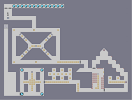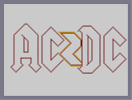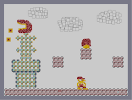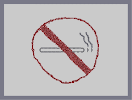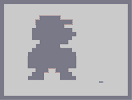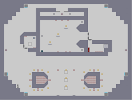WW2 escape AC/DC Super Mario No Smoking big mario (insert something clever)

## Comments

Pages: (0)

### nice job

looks just like the real thing

### hmmm

mrgy and ryzor both right on this one. it would look a lot better centered and slightly cleaned up - and with some colour/shading on it. oh and as a tip - on the II and some of the other parts - change to the c snap when doing the vertical and horizontal lines - makes it a lot nicer on the eye. z snap for everything thats not a straight line tho. so far N/R

### Wow.

I like it alot great job

### Doesn't

much look like the original...Look at the 'G' for instance...anyways, 2.5. not centered, crooked lines, and it's rather drab.

### Good so far

But don't stop here. You have alot of empty space, fill it up with something, music notes, abstract detail, something.